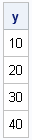In a previous blog, I showed how to use SAS/IML subscript reduction operators to compute the location of the maximum values for each row of a matrix. The subscript reduction operators are useful for computing simple statistics for each row (or column) of a numerical matrix.

If x is a matrix, I primarily use subscript reduction operators to compute the following quantities:

• the row vector that contains the sum of the elements for each column of a matrix: x[+, ]
• the row vector that contains the mean of the elements for each column of a matrix: x[:, ]
• the column vector that contains the sum of the elements for each row of a matrix: x[, +]
• the column vector that contains the mean of the elements for each row of a matrix: x[, :]
I wrote a 2011 article in which I gave examples of each of these operations and encouraged SAS/IML programmers to use subscript reduction operators to avoid loops over rows or columns.

Recently a SAS/IML programmer contacted me about how to compute the maximum value of each row in a matrix. He sent the following program, which uses a DO loop and the MAX function to compute a column vector whose ith element is the maximum value of the ith row of a matrix:

```proc iml; x = {10 0 1 0 2 0 4, 0 3 9 7 20 8 8, 4 4 30 9 0 2 1, 0 1 2 4 6 40 3 };   /* Find max of each row. Method 1: DO loop (inefficient) */ y = J(nrow(x), 1, 0); do i = 1 to nrow(x); y[i] = max(x[i, ]); end; print y;```You can eliminate the DO loop by using the <> operator, as follows:

```/* Method 2: subscript operator (efficient) */ y = x[, <>]; /* max of each row */```

The expression x[, <>] is read as follows:

• No subscripts are specified for the row index (before the comma). This means "use all rows." (You could also use the expression x[1:nrow(x), <>], but this is less efficient.)
• The operator (<>) is specified for the column index (after the comma). This means "find the maximum element for columns." Because the operator is specified in place of a column index, the result is a column vector.

The hardest part, for me, is remembering where to put the subscript reduction operator. I use the following mnemonics:

• If you want a column vector, use the operator in place of a column index: x[, <>]
• If you want a row vector, use the operator in place of a row index: x[<>, ]
• If you want a scalar value, use the operator as a sole subscript. For example, x[<>] computes the maximum element of an entire matrix, and is equivalent to max(x).

In addition to finding sums, means, maxima, and minima, you can also use subscript reduction operators to compute products (#) and sum of squares (##). These operations are useful for forming simple statistics for each row or for each column of a matrix.

Share1.